# How Current P&L is Calculated

We commonly get questions on why the Current P&L is not as expected (especially when foreign currencies are involved), and this page explains how StocksCafe calculates your Current P&L.

Here is an example portfolio (find your own here):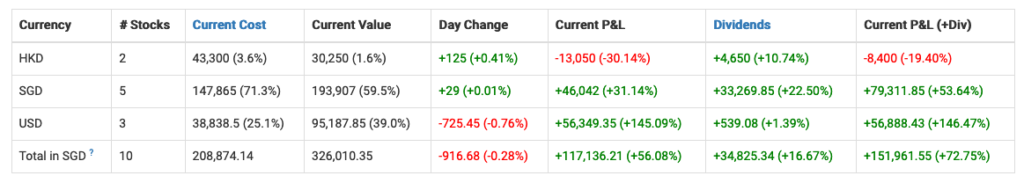Example portfolio

## The common (but incorrect) way of calculating Current P&L (Total in SGD)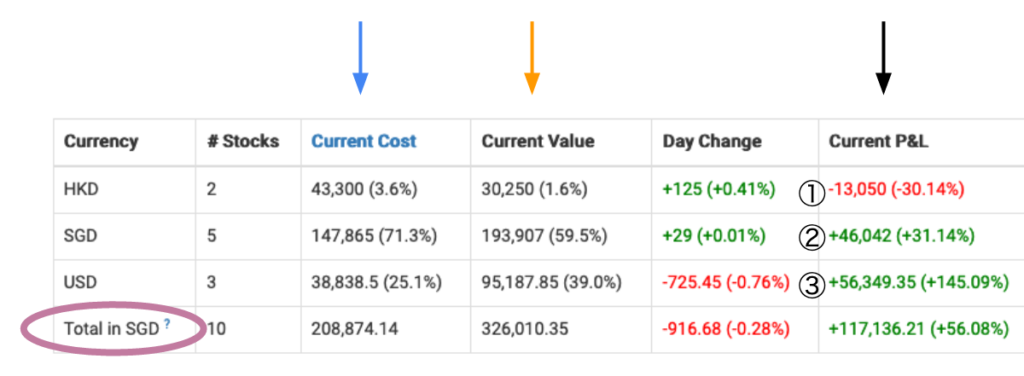Look at the Current P&L column

When there are transactions in foreign currencies, many people often just convert each currency’s Current P&L to SGD and add them up:

① x HKD exchange rate for today + ② + ③ x USD exchange rate for today

This is not how StocksCafe calculates Current P&L (Total in SGD).

## How StocksCafe calculates Current P&L (Total in SGD)

The formula is:

Current P&L for Total in SGD = Current Value for Total in SGD – Current Cost for Total in SGD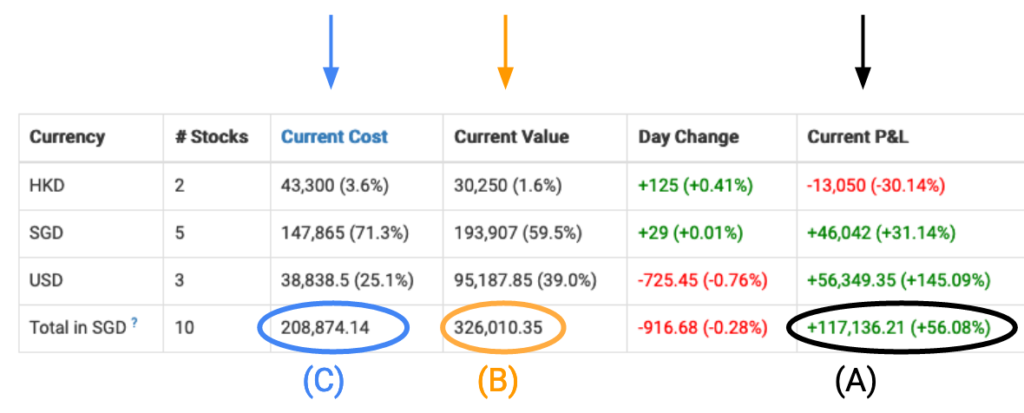Look at Total in SGD row

Simply put: (A) = (B) – (C).

## How to Calculate (B)

Current Value (B) for Total in SGD = Sum of Current Value in different currencies converted with the latest FX rate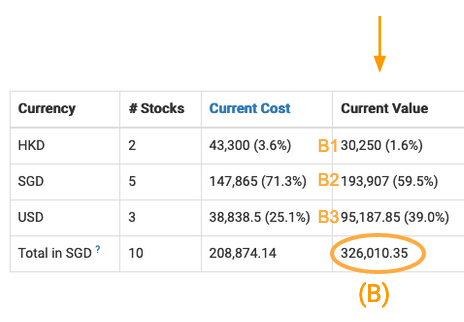Look at the Current Value column

Current Value (B) for Total in SGD =
B1 x latest HKD exchange rate
+ B2
+ B3 x latest USD exchange rate

## How to Calculate (C)

Current Cost (C) for Total in SGD = Sum of Cost for each transactions in their respective currencies and converted with the FX rate during the date of transactions (the FX rate could also be input by user)

Calculations for C are not so straightforward and rather tedious (but fortunately StocksCafe does it for you!)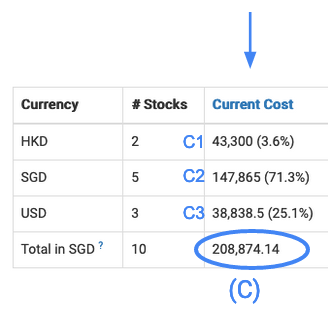Look at the Current Cost column

Take for example:

• There are a total of 2 transactions for HKD, one on 1 March 2020, one on 1 October 2020.
• There are a total of 3 transactions for USD, on 1, 2, 3 July 2020 respectively.

Current Cost (C) for Total in SGD =
Cost (in HKD) of 1 March transaction x HKD exchange rate on 1 March
+ Cost (in HKD) of 1 October transaction x HKD exchange rate on 1 October
+ C2
+ Cost (in USD) of 1 July transaction x USD exchange rate on 1 July
+ Cost (in USD) of 2 July transaction x USD exchange rate on 2 July
+ Cost (in USD) of 3 July transaction x USD exchange rate on 3 July

And there you have it, the breakdown of how StocksCafe calculates your Current P&L. If you have any further questions, feel free to comment below.